Courses

# Flanged Beams - Numerical Problems (Part -1) Civil Engineering (CE) Notes | EduRev

## Civil Engineering (CE) : Flanged Beams - Numerical Problems (Part -1) Civil Engineering (CE) Notes | EduRev

The document Flanged Beams - Numerical Problems (Part -1) Civil Engineering (CE) Notes | EduRev is a part of the Civil Engineering (CE) Course RCC & Prestressed Concrete.
All you need of Civil Engineering (CE) at this link: Civil Engineering (CE)

Instructional Objectives: At the end of this lesson, the student should be able to:

• identify the two types of numerical problems – analysis and design types,
• apply the formulations to analyse the capacity of a flanged beam,
• determine the limiting moment of resistance quickly with the help of tables of SP-16.

5.11.1 Introduction

Lesson 10 illustrates the governing equations of flanged beams. It is now necessary to apply them for the solution of numerical problems. Two types of numerical problems are possible: (i) Analysis and (ii) Design types.

This lesson explains the application of the theory of flanged beams for the analysis type of problems. Moreover, use of tables of SP-16 has been illustrated to determine the limiting moment of resistance of sections quickly for the three grades of steel. Besides mentioning the different steps of the solution, numerical examples are also taken up to explain their step-by-step solutions.

5.11.2   Analysis Type of Problems

The dimensions of the beam  bf, bw, Df, d, D, grades of concrete and steel and the amount of steel  Ast are given. It is required to determine the moment of resistance of the beam.

Step 1:  To determine the depth of the neutral axis  xu

The depth of the neutral axis is determined from the equation of equilibrium C =  T. However, the expression of C depends on the location of neutral axis, Df /d  and Df /xu parameters. Therefore, it is required to assume first that the xu is in the flange. If this is not the case, the next step is to assume xu in the web and the computed value of xu will indicate if the beam is underreinforced, balanced or over-reinforced.

Other steps: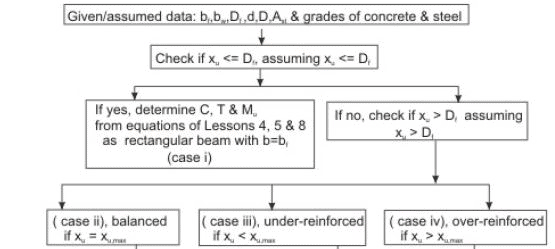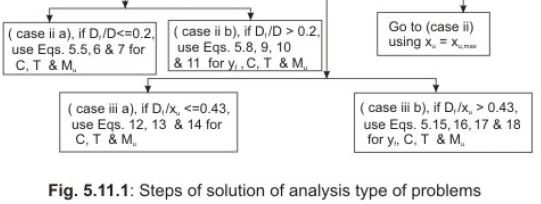After knowing if the section is under-reinforced, balanced or overreinforced, the respective parameter  Df/d or Df/Xis computed for the underreinforced, balanced or over-reinforced beam. The respective expressions of C, T and Mu, as established in Lesson 10, are then employed to determine their values. Figure 5.11.1 illustrates the steps to be followed.

5.11.3 Numerical Problems (Analysis Type)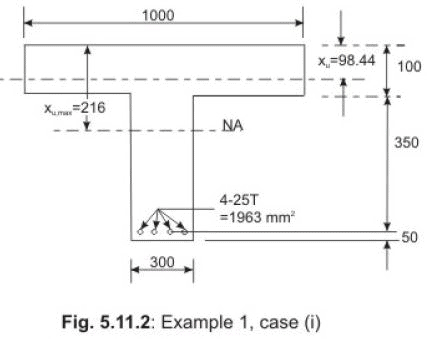Ex.1:  Determine the moment of resistance of the T-beam of Fig. 5.11.2. Given data: b =  1000 mm, Df  =  100 mm,  b =  300 mm,  cover  =  50 mm, d  =  450 mm and Ast  =  1963 mm2 (4- 25 T). Use M 20  and   Fe 415.

Step 1:  To determine the depth of the neutral axis xu

Assuming xu  in  the flange and equating total compressive and tensile forces from the expressions of  C  and  T  (Eq. 3.16 of Lesson 5) as the T-beam can be treated as rectangular beam of width bf  and effective depth  d, we get: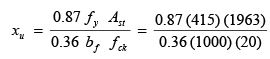= 98.44  mm  < 100 mm

So, the assumption of  xu  in the flange is correct. xu, max  for the balanced rectangular beam  =  0.48 d  =  0.48 (450)  =  216 mm.
It is under-reinforced since xu  <  xu,max.

Step 2:  To determine  C, T  and Mu

From Eqs. 3.9 (using b = bf) and 3.14 of Lesson 4 for C and T and Eq. 3.23 of Lesson 5 for Mu, we have:

C  =  0.36  b xu  fck          (3.9)

=  0.36 (1000) (98.44) (20)  =  708.77  kN

T  =  0.87  fy  Ast (3.14)

=  0.87 (415) (1963)  =  708.74 kN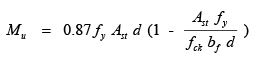(3.23)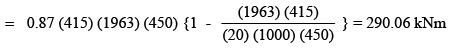This problem belongs to the case (i) and is explained in sec. 5.10.4.1 of Lesson 10.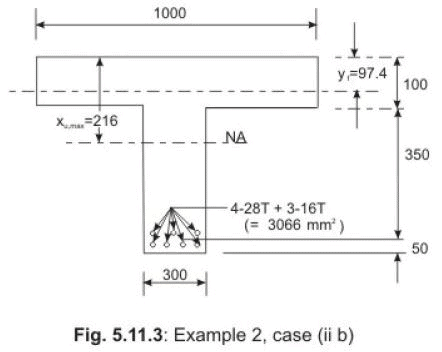Ex.2:  Determine  Ast,lim  and  Mu,lim  of the flanged beam of Fig. 5.11.3. Given data are: bf  =  1000 mm, D =  100 mm,  bw  =  300 mm,  cover  =  50 mm and  d =  450 mm. Use M 20  and   Fe 415.

Step 1:  To determine Df/d  ratio  For the limiting case xu  =  xu,max  =  0.48 (450)  =  216  mm  > Df.  The ratio Df/d  is computed.

Df/d   =  100/450  =  0.222  >  0.2 Hence, it is a problem of case (ii b) and discussed in sec. 5.10.4.2 b of Lesson 10.

Step 2:  Computations of  yf , C and T

First, we have to compute y from Eq.5.8 of Lesson 10 and then employ Eqs. 5.9, 10 and 11  of Lesson 10 to determine C, T  and  Mu, respectively.

yf  = 0.15 xu,max +  0.65 D =  0.15 (216) + 0.65 (100)

=  97.4 mm. (from Eq. 5.8)

C  =  0.36  fck  bw  xu,max  +  0.45  fck (b  -  bw) yf (5.9)

=  0.36 (20) (300) (216)  +  0.45 (20) (1000 - 300) (97.4) = 1,080.18 kN.

T  =  0.87  f Ast

=  0.87 (415) Ast (5.10)

Equating  C  and  T, we have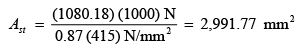Provide 4-28 T (2463 mm2) +  3-16 T (603  mm2)  =  3,066  mm

Step 3:  Computation of  Mu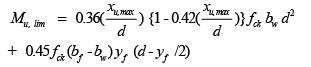(5.11)

=  0.36 (0.48) {1 - 0.42 (0.48)} (20) (300) (450)2
+ 0.45 (20) (1000 - 300) (97.4) (450 - 97.4/2) = 413.87 kNm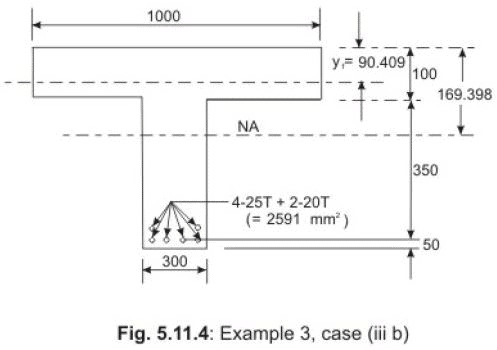Ex.3:  Determine the moment of resistance of the beam of Fig. 5.11.4 when  Ast = 2,591 mm2 (4- 25 T and 2- 20 T). Other parameters are the same as those of

Ex.1: b =  1,000 mm, D =  100 mm,  bw  =  300 mm,  cover  =  50 mm and d  =  450 mm. Use M 20  and   Fe 415.

Step 1:  To determine  xu

Assuming xu  t o  be in the flange and the beam is under-reinforced, we have from Eq. 3.16 of Lesson 5: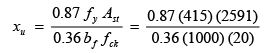=  129.93  mm  > 100  mm

Since x> Df, the neutral axis is in web.  Here, Df/d  =  100/450  =  0.222  >  0.2. So, we have to substitute the term y from Eq. 5.15 of Lesson 10, assuming Df / x >  0.43 in the equation of  C = T  from Eqs. 5.16 and 17 of  sec. 5.10.4.3 b of Lesson 10. Accordingly, we get:  0.36 fck  bw  xu  +  0.45  fck (bf   -  bw) yf  =  0.87 fy Ast

or 0.36 (20) (300) (xu) + 0.45 (20) (1000 - 300) {0.15 xu + 0.65 (100)}   =  0.87 (415) (2591)

or xu   =  169.398  mm  <  216 mm (xu,max = 0.48 xu = 216 mm)

So, the section is under-reinforced.

Step 2:  To determine  Mu

D/x =  100/169.398  =  0.590  >  0.43 This is the problem of case (iii b) of sec. 5.10.4.3 b. The corresponding equations are Eq. 5.15 of Lesson 10 for  y and Eqs. 5.16 to 18 of Lesson 10 for  C,  T and Mu, respectively. From Eq. 5.15 of Lesson 10, we have:

y =  0.15 xu +  0.65  D =  0.15 (169.398) + 0.65 (100)  =  90.409  mm

From Eq. 5.18 of Lesson 10, we have

M =  0.36(xu /d){1 - 0.42( xu /d)} fck bw d2 + 0.45  fck(bf - bw) yf (d - y/2)

or Mu  =  0.36 (169.398/450) {1 - 0.42 (169.398/450)} (20) (300) (450) (450) + 0.45 (20) (1000 - 300) (90.409) (450 - 90.409/2)

=  138.62 + 230.56  =  369.18  kNm.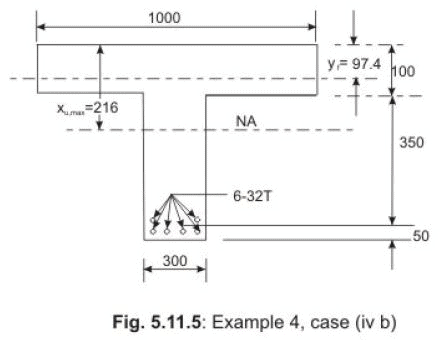Ex.4:  Determine the moment of resistance of the flanged beam of Fig. 5.11.5 with Ast = 4,825 mm(6- 32 T). Other parameters and data are the same as those of Ex.1: bf  =  1000 mm, Df  =  100 mm,  b =  300 mm,  cover  =  50 mm and d  =  450 mm. Use M 20  and   Fe 415.

Step 1:  To determine  xu

Assuming xu in  the flange of under-reinforced rectangular beam we have from Eq. 3.16 of Lesson 5: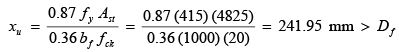Here, Df/d  =  100/450  =  0.222  >  0.2. So, we have to determine  yf  from Eq. 5.15 and equating  C and  T from Eqs. 5.16 and 17 of Lesson 10.

yf  =  0.15 xu +  0.65  Df       (5.15)

0.36 fck  bw  xu  +  0.45  fck (b  -  bw) yf  =  0.87 fy Ast            (5.16 and 5.17)

or 0.36 (20) (300) (xu) + 0.45 (20) (1000 - 300) {0.15 xu + 0.65 (100)}   =  0.87 (415) (4825)

or 2160 xu  +   945 x =  -  409500  + 1742066

or xu   =  1332566/3105  =  429.17 mm

xu,max  =  0.48 (450)  =  216 mm

Since  xu   >  xu,max, the beam is over-reinforced.

Accordingly. xu   =  xu, max   =  216 mm.

Step 2:  To determine  Mu

This problem belongs to case (iv b), explained in sec.5.10.4.4 b of Lesson 10.

So, we can determine Mu  from Eq. 5.11 of Lesson 10.

M =  0.36(xu, max /d){1 - 0.42(xu, max /d)} fck bw d2 + 0.45  fck(bf - bw) yf (d - yf /2)             (5.11)

where y =  0.15 xu, max +  0.65  Df    =  97.4  mm     (5.8)

From Eq. 5.11, employing the value of  y = 97.4 mm, we get:  Mu  =  0.36 (0.48) {1 - 0.42 (0.48)} (20) (300) (450) (450)

+ 0.45 (20) (1000 - 300) (97.4) (450 - 97.4/2)

=  167.63 + 246.24  =  413.87  kNm

It is seen that this over-reinforced beam has the same  Mu  as that of the balanced beam of Example 2.

5.11.4   Summary of Results of Examples 1-4

The results of four problems (Exs. 1-4) are given in Table 5.1 below. All the examples are having the common data except  Ast.

Table 5.1  Results of Examples 1-4 (Figs. 5.11.2 – 5.11.5)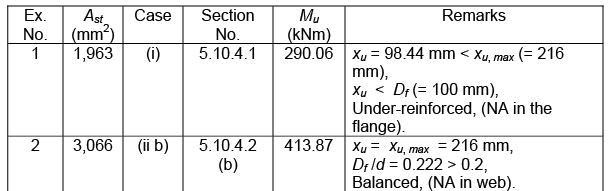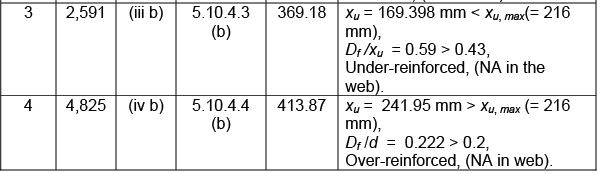It is clear from the above table (Table 5.1), that Ex.4 is an over-reinforced flanged beam. The moment of resistance of this beam is the same as that of balanced beam of Ex.2. Additional reinforcement of 1,759 mm2 (= 4,825 mm2 – 3,066 mm2) does not improve the Mof the over-reinforced beam. It rather prevents the beam from tension failure. That is why over-reinforced beams are to be avoided. However, if the Mu has to be increased beyond 413.87 kNm, the flanged beam may be doubly reinforced.

5.11.5  Use of SP-16 for the Analysis Type of Problems

Using the two governing parameters (bf /bw) and (Df /d), the Mu,lim of balanced flanged beams can be determined from Tables 57-59 of SP-16 for the three grades of steel (250, 415 and 500). The value of the moment coefficient Mu,lim /bwd2fck of Ex.2, as obtained from SP-16, is presented in Table 5.2 making linear interpolation for both the parameters, wherever needed. Mu,lim  is then calculated from the moment coefficient.

Table 5.2 Mu,lim of Example 2 using Table 58 of SP-16

Parameters:  (i)  bf /b =  1000/300  =  3.33         (ii) Df /d   =  100/450  =  0.222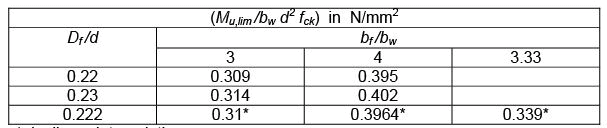by linear interpolation

So, from Table 5.2,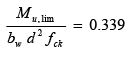Mu,lim  =  0.339 bd2 fck  =  0.339 (300) (450) (450) (20) 10-6  =  411.88 kNm

Mu,lim as obtained from SP-16 is close to the earlier computed value of Mu,lim = 413.87 kNm (see Table 5.1).

5.11.6 Practice Questions and Problems with Answers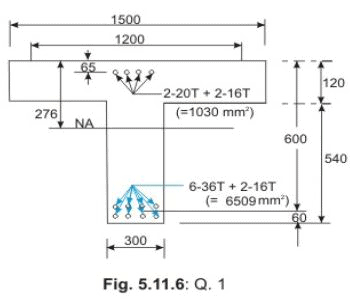Q.1:  Determine the moment of resistance of the simply supported doubly reinforced flanged beam (isolated) of span 9 m as shown in Fig. 5.11.6. Assume M 30 concrete and Fe 500 steel.
A.1:
Solution of Q.1:
Effective width  bf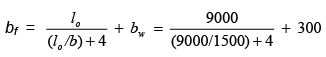= 1200  mm

Step 1:  To determine the depth of the neutral axis

Assuming neutral axis to be in the flange and writing the equation  C = T, we have:  0.87 fy Ast   =  0.36 fck bf xu  +  (fsc Asc – fcc Asc) Here, d ' / d  =  65/600  =  0.108 = 0.1 (say). We, therefore, have fsc  =  353 N/mm.  From the above equation, we have: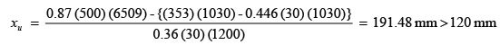So, the neutral axis is in web. Df /d  = 120/600  =  0.2 Assuming  Df /xu  <  0.43, and  Equating  C = T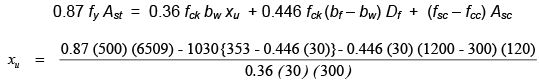= 319.92 >  276 mm (  = 276 mm)

So,  xu  =  xu,max  =  276 mm  (over-reinforced beam). Df /x =  120/276  =  0.4347  >  0.43

Let us assume  Df /xu > 0.43. Now, equating  C = T with  y  as the depth of flange having constant stress of 0.446 fck. So, we have:

yf  =  0.15 xu + 0.65 D =  0.15 x+ 78  0.36 fck bw  xu + 0.446 fck (b– bw) yf + Asc (fsc – fcc) = 0.87 fy Ast

0.36 (30) (300) x+ 0.446 (30) (900) (0.15 xu + 78)  =  0.87 (500) (6509) – 1030 {353 – 0.446 (30)}

or xu  =  305.63 mm >  xu,max.   (xu,max  =  276 mm)

The beam is over-reinforced. Hence,  xu  =  xu,max  = 276 mm. This is a problem of case (iv), and we, therefore, consider the case (ii) to find out the moment of resistance in two parts: first for the balanced singly reinforced beam and then for the additional moment due to compression steel.

Step 2:  Determination of  xu,lim for singly reinforced flanged beam

Here, Df /d  =  120/600  =  0.2, so  yf  is not needed. This is a problem of case (ii a) of sec. 5.10.4.2 of Lesson 10.

Employing Eq. 5.7 of Lesson 10, we have:

Mu,lim  =  0.36 (xu,max /d) {1 – 0.42 (xu,max /d)} fck bw d2    + 0.45 fck (bf – bw) D(d – D/2)

=  0.36(0.46) {1 – 0.42(0.46)} (30) (300) (600) (600)
+  0.45(30) (900) (120) (540)
=  1,220.20  kNm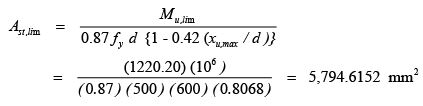Step 3:  Determination of  Mu2

Total Ast  =    6,509  mm2,  Ast,lim  =  5,794.62  mm2

Ast2  =  714.38  mm2  and  Asc  =  1,030  mm2

It is important to find out how much of the total Asc  and  Ast2  are required effectively. From the equilibrium of C and T  forces due to additional steel (compressive and tensile), we have:  (Ast2) (0.87) (fy)  =  (Asc) (fsc) If we assume  Asc  =  1,030 mm2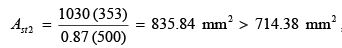(714.38 mm2 is the total Ast2 provided). So, this is not possible.  Now, using  Ast2 = 714.38 mm2 , we get Asc   from the above equation.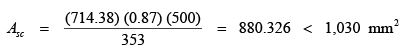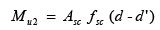(880.326 ) (1,030  mm2 sc 353 A == < , (1,030 mm2 is the total Asc provided). Mu2  =  Asc f sc (d - d ' )  =  (880.326) (353) (600 - 60)  =  167.807  kNm

Total moment of resistance  =  Mu,lim + Mu2  =  1,220.20 + 167.81  =  1,388.01  kNm

Total  Ast  required  =  Ast,lim + Ast2  =  5,794.62 + 714.38  =  6,509.00  mm2 , (provided Ast  = 6,509 mm2)

Asc required  =  880.326  mm (provided 1,030  mm2).

5.11.7  Test 11 with Solutions

Maximum Marks = 50,
Maximum Time = 30 minutes

TQ.1: Determine  Mu,lim  of the flanged beam of Ex. 2 (Fig. 5.11.3) with the help of SP-16 using (a) M 20 and Fe 250, (b) M 20 and Fe 500 and (c) compare the results with the Mu,lim  of Ex. 2 from Table 5.2 when grades of concrete and steel are M 20 and Fe 415, respectively. Other data are: bf  =  1000 mm, Df  =  100 mm,  b =  300 mm,  cover  =  50 mm and  d  =  450 mm.                           (10 X 3 = 30 marks)
A.TQ.
1: From the results of Ex. 2 of sec. 5.11.5 (Table 5.2), we have:

Parameters:  (i)  b/bw  =  1000/300  =  3.33    (ii) Df /d   =  100/450  =  0.222

For part (a):  When Fe 250 is used, the corresponding table is Table 57 of SP16. The computations are presented in

Table 5.3 below: Table 5.3  (Mu,lim /bw d2 fck)  in  N/mm2   Of TQ.1 (PART a for M 20 and Fe 250)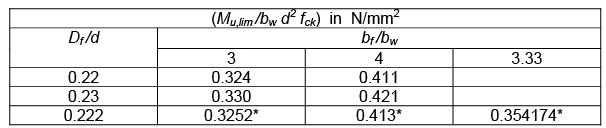by linear interpolation Mu,lim /bw dfck  = 0.354174 = 0.354 (say)

So, Mu,lim =  (0.354) (300) (450) (450) (20) N mm   = 430.11 kNm For part (b):  When Fe 500 is used, the corresponding table is Table 59 of SP16. The computations are presented in

Table 5.4 below: Table 5.4  (Mu,lim /bw d2 fck)  in  N/mm2   Of TQ.1 (PART b for M 20 and Fe 500)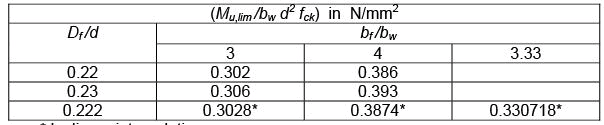* by linear interpolation

Mu,lim /bw d2 fck  = 0.330718 = 0.3307 (say)

So, Mu,lim =  (0.3307) (300) (450) (450) (20) mm   = 401.8  kNm

For part (c):  Comparison of results of this problem with that of Table 5.2 (M 20 and Fe    415) is given below in Table 5.5.

Table 5.5 Comparison of results of Mu,lim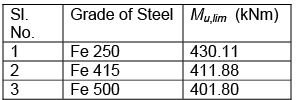It is seen that Mu,lim of the beam decreases with higher grade of steel for a particular grade of concrete.

TQ.2: With the aid of SP-16, determine separately the limiting moments of resistance and the limiting areas of steel of the simply supported isolated, singly reinforced and balanced flanged beam of

Q.1 as shown in Fig. 5.11.6 if the span =  9 m. Use M 30 concrete and three grades of steel, Fe 250, Fe 415 and Fe 500, respectively. Compare the results obtained above with that of

Q.1 of sec. 5.11.6, when balanced. (15 + 5  = 20 marks)
A.TQ.2: From the results of

Q.1 sec. 5.11.6, we have: Parameters:  (i)  b/bw  =  1200/300  =  4.0         (ii) D/d   =   120/600  =   0.2

For Fe 250, Fe 415 and Fe 500, corresponding tables are Table 57, 58 and 59, respectively of SP-16. The computations are done accordingly. After computing the limiting moments of resistance, the limiting areas of steel are determined as explained below. Finally, the results are presented in Table 5.6 below: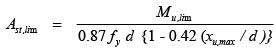Table 5.6 Values of Mu,lim  in  N/mm2   Of TQ.2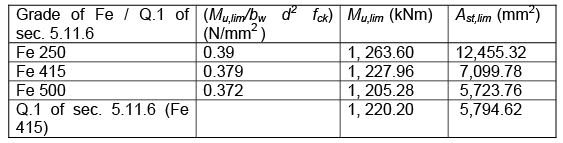The maximum area of steel allowed is .04 b D = (.04) (300) (660) = 7,920 mm2 . Hence, Fe 250 is not possible in this case.

Summary of this Lesson

This lesson mentions about the two types of numerical problems (i) analysis and (ii) design types. In addition to explaining the steps involved in solving the analysis type of numerical problems, several examples of analysis type of problems are illustrated explaining all steps of the solutions both by direct computation method and employing SP-16. Solutions of practice and test problems will give readers the confidence in applying the theory explained in Lesson 10  in solving the numerical problems.

Offer running on EduRev: Apply code STAYHOME200 to get INR 200 off on our premium plan EduRev Infinity!

## RCC & Prestressed Concrete

14 videos|24 docs|12 tests

,

,

,

,

,

,

,

,

,

,

,

,

,

,

,

,

,

,

,

,

,

;